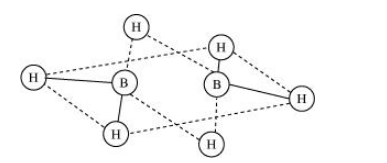# What characteristics do you expect from an electron-deficient hydride

Question:

What characteristics do you expect from an electron-deficient hydride with respect to its structure and chemical reactions?

Solution:

An electron-deficient hydride does not have sufficient electrons to form a regular bond in which two electrons are shared by two atoms e.g., $\mathrm{B}_{2} \mathrm{H}_{6}, \mathrm{Al}_{2} \mathrm{H}_{6}$ etc.

These hydrides cannot be represented by conventional Lewis structures. $\mathrm{B}_{2} \mathrm{H}_{6}$, for example, contains four regular bonds and two three centered-two electron bond. Its structure can be represented as:Since these hydrides are electron-deficient, they have a tendency to accept electrons. Hence, they act as Lewis acids.

$\mathrm{B}_{2} \mathrm{H}_{6}+2 \mathrm{NMe} \longrightarrow 2 \mathrm{BH}_{3} \cdot \mathrm{NMe}_{3}$

$\mathrm{B}_{2} \mathrm{H}_{6}+2 \mathrm{CO} \longrightarrow 2 \mathrm{BH}_{3} \cdot \mathrm{CO}$